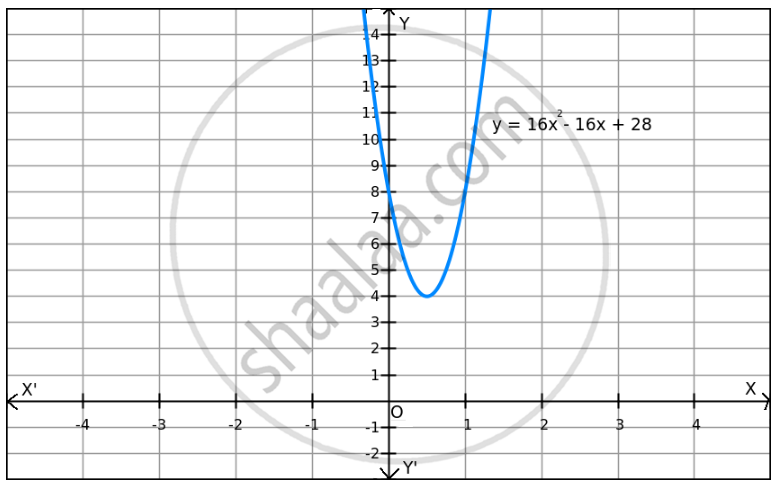Share

# F(X) = 16x2 $-$ 16x + 28 on R ? - Mathematics

#### Question

f(x) = 16x2 $-$ 16x + 28 on R ?

#### Solution

Given: f(x) = 16x2 − 16x + 28

$\Rightarrow$ f(x) = 4(4x2 - 4x + 1) + 24

$\Rightarrow$ f(x) = 4(2x − 1)2 + 24

Now,
4(2x − 1)$\geq$ 0 for all x $\in$ R

$\Rightarrow$ f(x) = 4(2x − 1)2 + 24 $\geq$ 24 for all x $\in$ R

$\Rightarrow$ f(x)$\geq$ 24 for all x $\in$ R.The minimum value of f is attained when (2x − 1) = 0.
(2x − 1) = 0

⇒ x = $\frac{1}{2}$

Therefore, the minimum value of f  at x =$\frac{1}{2}$ is 24.

Since f(x) can be enlarged, the maximum value does not exist, which is evident in the graph also.

Hence, the function f does not have a maximum value.

Is there an error in this question or solution?
F(X) = 16x2 $-$ 16x + 28 on R ? Concept: Graph of Maxima and Minima.